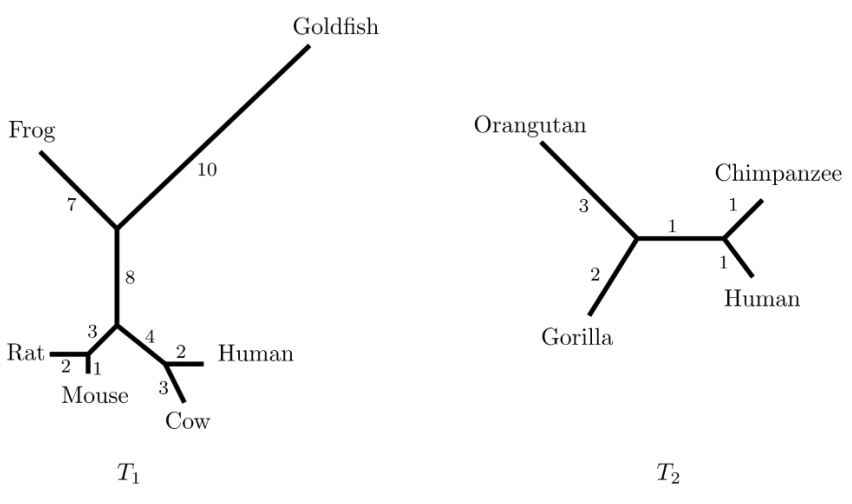시간 제한메모리 제한제출정답맞힌 사람정답 비율
1 초 512 MB33719314761.506%

## 문제

A gene tree is a tree showing the evolution of various genes or biological species. A gene tree represents the relatedness of specific genes stored at the leaf nodes without assumption about their ancestry. Leaf nodes represent genes, called taxa, and internal nodes represent putative ancestral taxa. Each edge in the tree is associated with a positive integer, phylogenetic length, which quantifies the evolutionary distance between two nodes of the edge. For example, the left figure below shows a gene tree with six leaf nodes, which approximates the relation among six taxa, and the right one shows a gene tree with four taxa.Figure B.1: Unrooted gene trees T1 and T2.

Like the trees T1 and T2 above, gene trees are modeled as unrooted trees where each internal node (non-leaf node) has degree three. A path-length between two leaf nodes is the sum of the phylogenetic lengths of the edges along the unique path between them. In T1, the path-length between Human and Cow is 2 + 3 = 5 and the path-length between Human and Goldfish is 2 + 4 + 8 + 10 = 24. These lengths indicate that Human is much closer to Cow than to Goldfish genetically. From T2, we can guess that the primate closest to Human is Chimpanzee.

Researchers are interested in measuring the distance between genes in the tree. A famous distance measure is the sum of squared path-lengths of all unordered leaf pairs. More precisely, such a distance d(T) is defined as follows:

$d(T) = \sum_{\text{unordered pair } (u, v)}{p^2_{u, v}}$

where pu,v is a path-length between two leaf nodes u and v in T. Note that d(T) is the sum of the squared path-lengths p2u,v over all unordered leaf pairs u and v in T. For the gene tree T2 in Figure B.1, there are six paths over all unordered leaf pairs, (Human, Chimpanzee), (Human, Gorilla), (Human, Orangutan), (Chimpanzee, Gorilla), (Chimpanzee, Orangutan), and (Gorilla, Orangutan). The sum of squared path-lengths is 22 + 42 + 52 + 42 + 52 + 52 = 111, so d(T2) = 111.

Given an unrooted gene tree T, write a program to output d(T).

## 입력

Your program is to read from standard input. The input starts with a line containing an integer n (4 ≤ n ≤ 100,000), where n is the number of nodes of the input gene tree T. Then T has n − 1 edges. The nodes of T are numbered from 1 to n. The following n − 1 lines represent n − 1 edges of T, where each line contains three non-negative integers a, b, and l (1 ≤ a ≠ b ≤ n, 1 ≤ l ≤ 50) where two nodes a and b form an edge with phylogenetic length l.

## 출력

Your program is to write to standard output. Print exactly one line. The line should contain one positive integer d(T).

## 예제 입력 1

4
1 4 1
4 3 1
2 4 1


## 예제 출력 1

12


## 예제 입력 2

6
1 5 1
5 2 1
5 6 1
6 4 3
6 3 2


## 예제 출력 2

111


## 예제 입력 3

10
1 2 10
10 2 7
3 2 8
3 9 3
9 8 2
7 9 1
6 4 3
4 5 2
3 4 4


## 예제 출력 3

4709